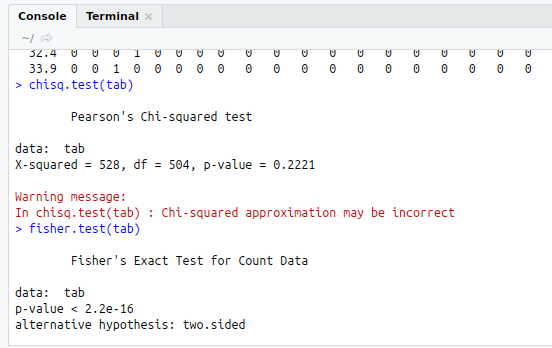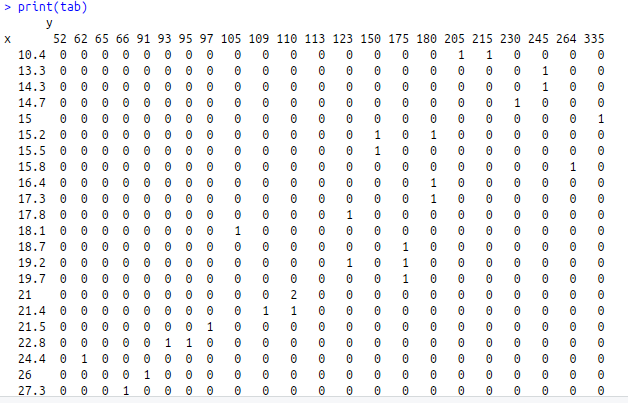• #5, First Floor, 4th Street Dr. Subbarayan Nagar Kodambakkam, Chennai-600 024 Landmark : Samiyar Madam
• pro@slogix.in
• +91- 81240 01111

How to test independence of variable using chi-squared test in R?

Description

To test the independence of variable using chi-squared test in R programming.

Chi- squared test :

To test independence of the row and column variable.

p-value is calculated from the asymptotic chi-squared distribution of the test statistic

H0 : Two Variables are independent.

H1 : Two Variables are relative.

R Function :test()

Fisher Test :

Provides an exact test of independence.

R Function :test(x)

x - two dimensional contingency table in matrix form.

Sapmle Code

#Independence test
#Chi-Squared Test
#Input
attach(mtcars)
x<-mtcars\$mpg
y<-mtcars\$hp

#Table Function
tab<-table(x,y)
print(tab)
chisq.test(tab)

#Fisher Test
fisher.test(tab)

Screenshots